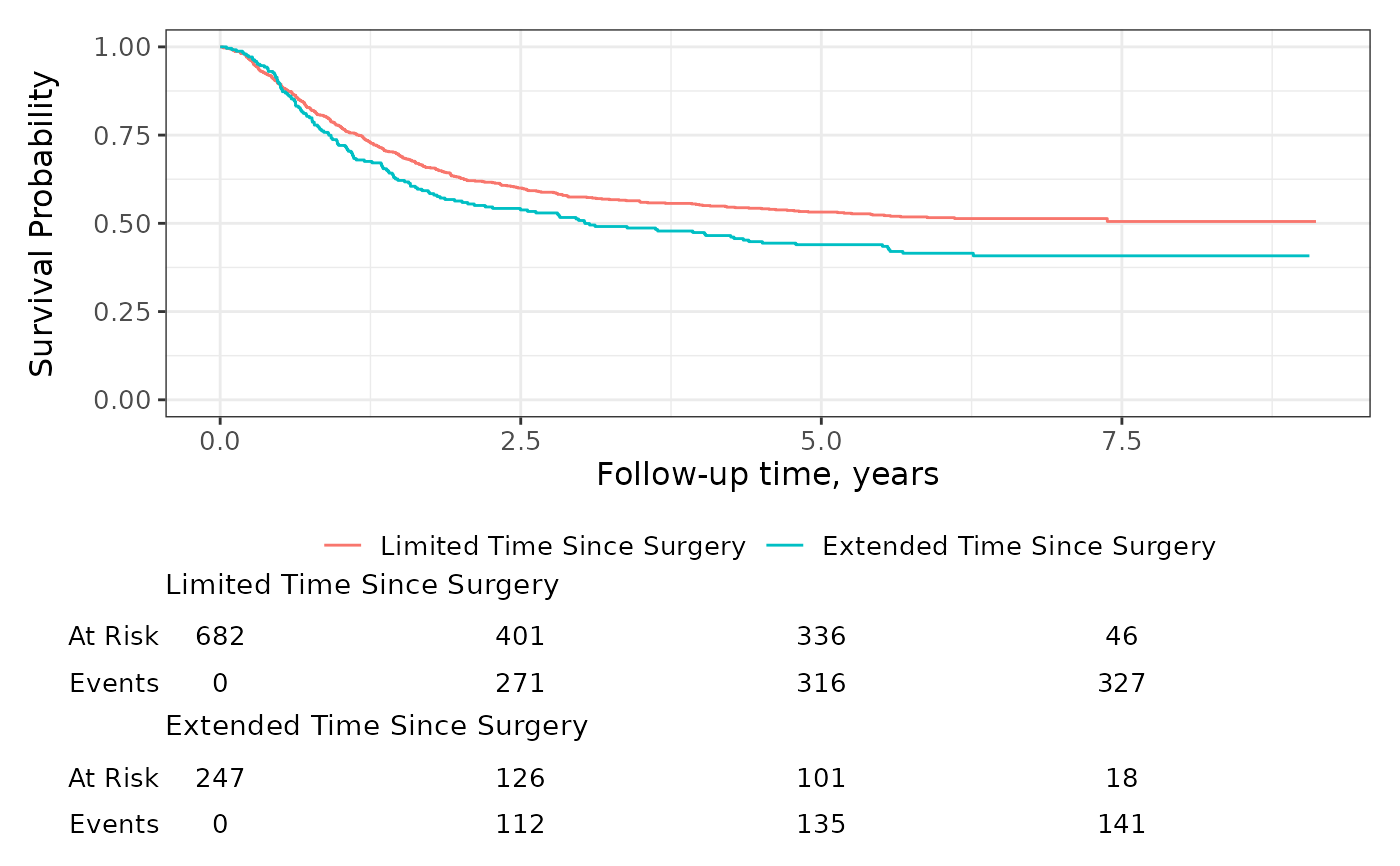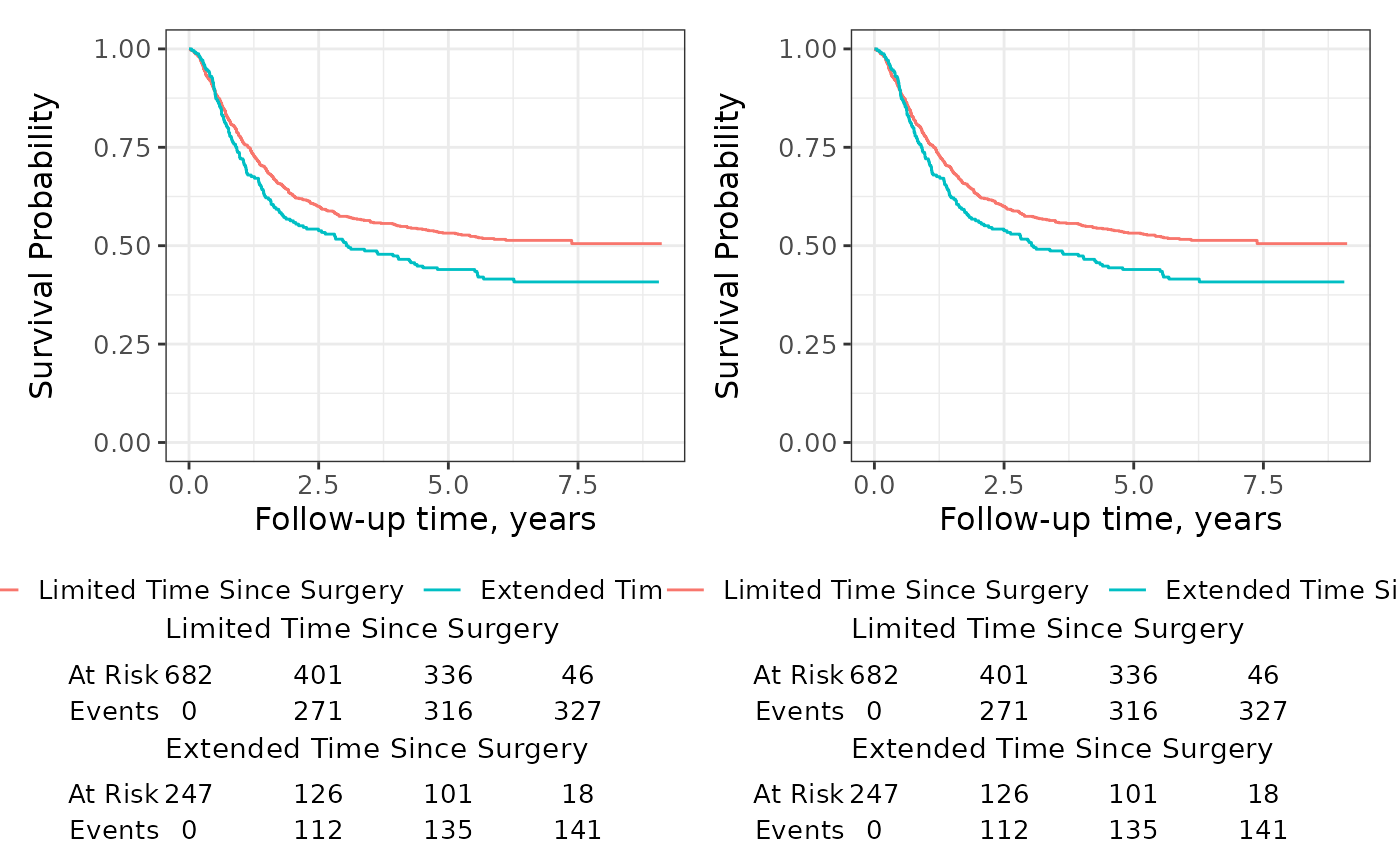Function takes an object created with ggsurvfit() or ggcuminc() and prepares the plot for printing. If a plot also has a risk table, this function will build the risk table plots and return them either as list of plots or combined using patchwork::wrap_plots().

This can be particularly useful when you would like to place figures with risk tables side-by-side.

## Usage

ggsurvfit_build(x, combine_plots = TRUE)

## Arguments

x

an object of class 'ggsurvfit' or 'ggcuminc'

combine_plots

logical indicating whether to combine the primary plot and the risk tables. When TRUE, plot and risk table(s) are combined with patchwork::wrap_plots(). When FALSE and the plot has risk tables, they are returned in a list of gtable grobs. Default is TRUE.

## Value

a list of ggplot2 objects or a single ggplot2 object

Visit the gallery for examples modifying the default figures

## Examples

# construct plot
p <-
survfit2(Surv(time, status) ~ surg, df_colon) %>%
ggsurvfit() +
scale_y_continuous(limits = c(0, 1))

# build plots
built_p <- ggsurvfit_build(p, combine_plots = FALSE)

# reconstruct original figure print with risktables
patchwork::wrap_plots(
built_p[],
built_p[],
built_p[],
ncol = 1,
heights = c(0.70, 0.15, 0.15)
)# place plots side-by-side (plots must be built before placement with patchwork)
patchwork::wrap_plots(
ggsurvfit_build(p),
ggsurvfit_build(p),
ncol = 2
)